# OliNo

Renewable Energy

## Lemnis – Pharox 300 Flame E27 ledlamp.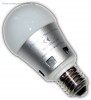presents an led light bulb that emits a deep warm white color. Three light bulbs are measured and this articles gives the average results as well as the deviations seen.

This article shows the measurement results. Many parameters are also found in the Eulumdat file.

See this overview for a comparison with other light bulbs.

### Summary measurement data

parameter meas. result remark
Color temperature 2018 K Deep warm white. Variation +63 and -37 K over three light bulbs.
Luminous intensity Iv 49 Cd Measured straight underneath the lamp. Variation 1, -3.
Beam angle 195 deg 195º for the all C-planes as the lamp has symmetry over its 1st axis. Variation +3, -3.
Power P 5.3 W Follow the link for more electrical and temperature properties. Variation +0.1, -0.
Power Factor 0.53 For every 1 kWh net power consumed, there has been 1.6 kVAhr for reactive power. Variation +0.03, -0.02.
Luminous flux 349 Lm Variation +7, -8.
Luminous efficacy 66 Lm/W Variation +0, -1.
CRI_Ra 91 Color Rendering Index. Variation +1, -1.
Coordinates chromaticity diagram x=0.5269 and y=0.4169
Fitting E27
PAR-value 0.5 μMol/s/m2 The number of photons seen by an average plant when it is lit by the light of this light bulb. Value valid at 1 m distance from light bulb. Variation +0, -0.1.
PAR-photon efficacy 0.6 μMol/s/We The toal emitted number of photons by this light, divided by its consumption in W. It indicates a kind of efficacy in generating photons. Variation0.
S/P ratio 0.9 This factor indicates the amount of times more efficient the light of this light bulb is perceived under scotopic circumstances (ow environmental light level). Variation 0.
D x H external dimensions 59 x 116 mm External dimensions of the lamp (D = diameter).
D x H luminous area 59 x 42 mm Dimensions of the luminous area (used in Eulumdat file). This is equal to the surface of matte white glass.
General remarks The ambient temperature during the whole set of measurements was 23-24.5 deg C. The maximum temperature of the light bulb gets about 29 degrees hotter than ambient. Variation +1.5, -1 deg C.
Warm up effect: during the warm up time the illuminance decreases with 21 % and the consumed power with 8 %.

Voltage dependency: the power consumption and illuminance do not significantly depend on the voltage when it is varied from 200 – 250 V.

This article contains the test results of dimming the lamp at the end, which works well with the by OliNo used dimmer.

Measurement report (PDF)Eulumdat fileRight click on icon and save the file.
IES fileRight click on icon and save the file.

### Overview table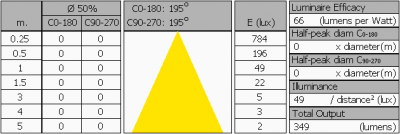The overview table is explained on the OliNo website. Please note that this overview table makes use of calculations, use this data with care as explained on the OliNo site. E (lux) values are not accurate, when within 5 x 59 mm ≈ 360 mm. Within this distance from the lamp, the measured lux values willl be less than the computed values in this overview as the measurements are then within the near field of the lamp.

Note also that the beam angle > 180 degrees, which means that the spot sizes of Ø50cm cannot be computed and the reported values should be discarded.

### Eulumdat light diagram

This light diagram below comes from the program Qlumedit, that extracts these diagrams from an Eulumdat file. It is explained on the OliNo site.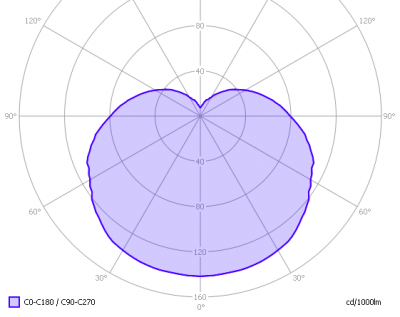The light diagram giving the radiation pattern.

It indicates the luminous intensity around the light bulb. In all C-planes the diagram is the same due to its symmetry along its 1st axis.

### Illuminance Ev at 1 m distance, or luminous intensity Iv

Herewith the plot of the averaged luminous intensity Iv as a function of the inclination angle with the light bulb.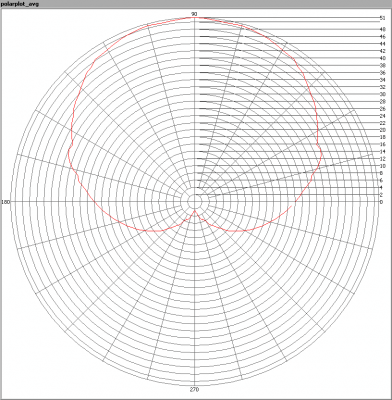The radiation pattern of the light bulb.

This radiation pattern is the average of the light output of the light diagram given earlier. Also, in this graph the luminous intensity is given in Cd.

These averaged values are used (later) to compute the lumen output.Intensity data of every measured turn angle at each inclination angle.

This plot shows per inclination angle the intensity measurement results for each turn angle at that inclination angle. There normally are differences in illuminance values for different turn angles. However for further calculations the averaged values will be used.

When using the average values per inclination angle, the beam angle can be computed, being 195º.

### Luminous flux

With the averaged illuminance data at 1 m distance, taken from the graph showing the averaged radiation pattern, it is possible to compute the luminous flux.

The result of this computation for this light spot is a luminous flux of 349 Lm.

### Luminous efficacy

The luminous flux being 349 Lm, and the power of the light bulb being 5.3 W, yields a luminous efficacy of 66 Lm/W.

### Electrical properties

A power factor of 0.53 means that for every 1 kWh net power consumed, a reactive component of 1.6 kVAr was needed.

 Lamp voltage 230 VAC Lamp current 43 mA Power P 5.3 W Apparent power S 10.0 VA Power factor 0.53

Of this light bulb the voltage across ad the resulting current through it are measured and graphed. See the OliNo site how this is obtained.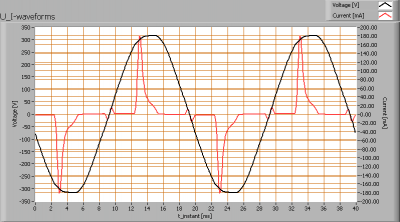Voltage across and current through the lightbulb

This waveforms have been checked on requirements posed by the norm IEC 61000-3-2:2006 (including up to A2:2009). See also the explanation on the OliNo website.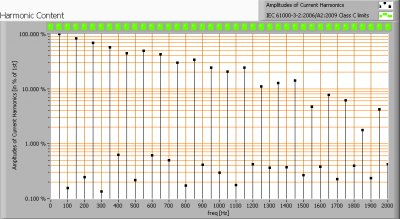Harmonics in in the current waveform and checked against IEC61000-3-2:2006

There are no limits for the harmonics for led lighting equipment <= 25 W.

The Total Harmonic Distortion of the current is computed as 159 %.

### Temperature measurements lamp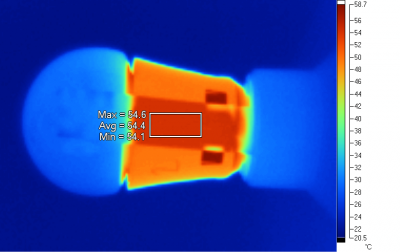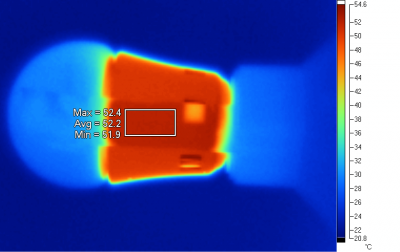Temperature overview images of lamps 1, 2 and 3. Tape is used to start from a known emissivity.

 status lamp > 2 hours on ambient temperature 24 deg C reflected background temperature 24 deg C camera Fluke Ti25 emissivity 0.95(1) measurement distance 0.30 m IFOVgeometric 0.8 mm NETD (thermal sensitivity) 100 mK

(1) The emissivity of the masking tape is used which is about 0.95. The masking tape is so thin that it quickly takes the same temperature as the light bulb’s heat sink.

The basis of the light bulb on its hotttest side gets to about 53 deg C. This is on the lamp’s base.

### Color temperature and Spectral power distribution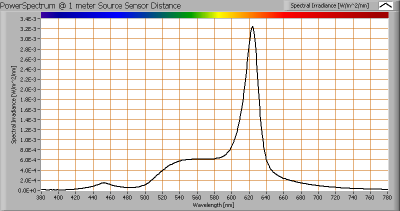The spectral power distribution of this light bulb, energies on y-axis valid at 1 m distance.

The measured color temperature is about 2018 K which is deep warm white.

This color temperature is measured straight underneath the light bulb. Below a graph showing the color temperature for different inclination angles.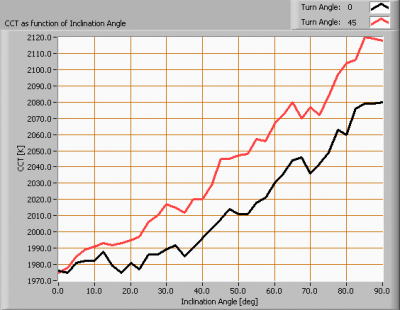Color temperature as a function of inclination angle.

The measurement of CCT is measured for inclination angles up to 90º.

The beam angle for the C0-C180 is 195º, meaning a 97.5º inclination angle. In this area the majority of the light is present. The variation in correlated color temperature in the first 90 degrees inclination area is ≈ 7 %.

### PAR value and PAR spectrum

To make a statement how well the light of this light bulb is for growing plants, the PAR-area needs to be determined. See the OliNo website how this all is determined and the explanation of the graph.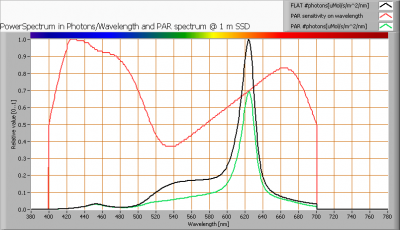The photon spectrum, then the sensitivity curve and as result the final PAR spectrum of the light of this light bulb

parameter value unit
PAR-number 0.5 μMol/s/m²
PAR-photon current 3.1 μMol/s
PAR-photon efficacy 0.6 μMol/s/W

The PAR efficiency is 63 % (valid for the PAR wave length range of 400 – 700 nm). So maximally 63 % of the total of photons in the light is effectively used by the average plant (since the plant might not take 100 % of the photons at the frequency where its relative sensitivity is 100 %).

### S/P ratio

The S/P ratio and measurement is explained on the OliNo website. Here the results are given.The power spectrum, sensitivity curves and resulting scotopic and photopic spectra (spectra energy content defined at 1 m distance).

The S/P ratio is 0.9.

More info on S/P ratio can be found on the OliNo website.

### Chromaticity diagram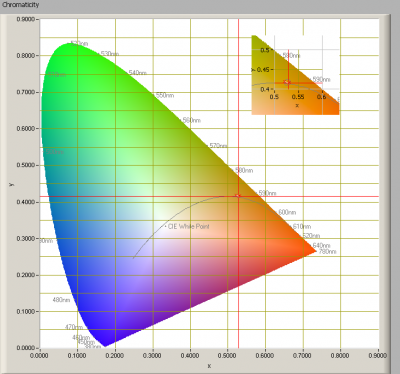The chromaticity space and the position of the lamp’s color coordinates in it.

The light coming from this lamp is close at the Planckian Locus (the black path in the graph).

Its coordinates are x=0.5269 and y=0.4169. The color coordinates of the three tested lamps are within the 4 steps McAdams ellipse.

### Color Rendering Index (CRI) or also Ra

Herewith the image showing the CRI as well as how well different colors are represented (rendered). The higher the number, the better the resemblance with the color when a black body radiator would have been used (the sun, or an incandescent lamp). Practical information and also some critics about the CRI can be found on the OliNo website.

Each color has an index Rx, and the first 8 indexes (R1 .. R8) are averaged to compute the Ra which is equivalent to the CRI.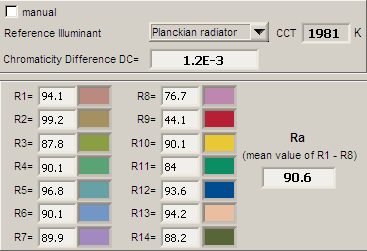CRI of the light of this lightbulb.

The value of 91 is higher than 80 which is considered a minimum value for indoor usage.

Note: the chromaticity difference is 0.0012 indicates the distance to the Planckian Locus. There is no norm yet that states what the max deviation from white light is allowed to be.

### Voltage dependency

The dependency of a number of lamp parameters on the lamp voltage is determined. For this, the lamp voltage has been varied and its effect on the following light bulb parameters measured: illuminance E_v [lx], the lamppower P [W], the (Correlated) Color Temperature [K] and the luminous efficacy [Lm/W].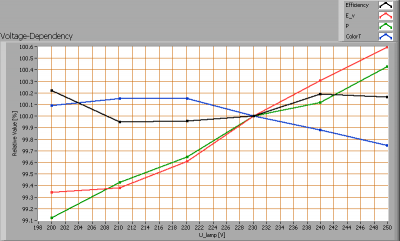Lamp voltage dependencies of certain light bulb parameters, where the value at 230 V is taken as 100 %.

The illuminance and consumed power do not (significantly) vary when the voltage is varied, in a linear matter.

When the voltage at 230 V varies with + and – 5 V, then the illuminance varies < 0.25 %, so when abrupt voltage changes occur this effect is not visible in the illuminance output.

### Warm up effects

After switch on of a cold lamp, the effect of heating up of the lamp is measured on illuminance E_v [lx], color temperature CT or correlated color temperature CCT [K], the lamppower P [W] and the luminous efficacy [lm/W].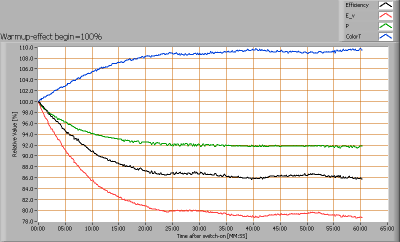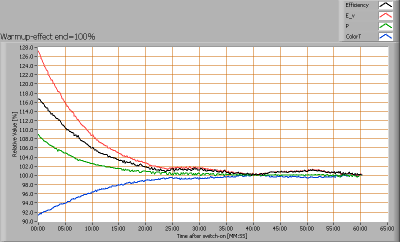Effect of warming up on different light bulb parameters. At top the 100 % level is put at begin, and at bottom at the end.

The warm up time is about 25 minutes. During that time the illuminance decreases 12 % and the consumed power with 6 %.

### Dim function

The lamp has been measured on its ability to be dimmed. See the following graph that gives the result of the dimming function. The general interpretation of the parameters and the graph and the way the dimming function is tested is explained on the OliNo website.

The dim-test is done with the OliNo dimmer (Elimpo brand).

This dimmer is used at 50 different positions, from 100 % (no dimming) to 0 % (maximal dimming). The effect of dimming on some lamp parameters is measured and determined.

The initial illuminance loss when a dimmer like the Elimpo is inserted, and when put to no dimming at all, is determined and found to be 1 %.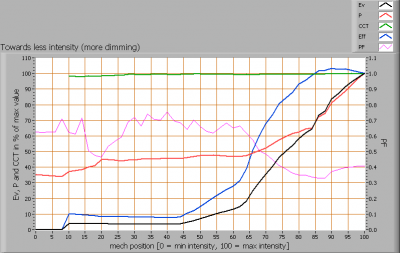Lamp parameter values dependent on the dim position

Mechanical range for dimming: from 40 – 100 %, this is a wide range.

Illuminance/Intensity value range: from 0 – 100 %, the total range.

Consumed power decreases about as fast as the illuminance value decreases. At the end the consumed power remains constant while the illuminance still decreases, which can also be due to the used dimmer.

The color temperature stays constant.

The powerfactor at no dimming is 0.4 which is bit lower than without dimmer. When dimming is increased the power factor even increases.